# Maths - Concrete Category - Topology

There are various categories associated with topology. The most fundamental is Top - Category of Topological Spaces. There is a category of the subset structure of open sets. There are also categories of fibres and sheaves.

## Top - Topological Spaces

Consider a category C as follows:

• Objects are spaces
• Morphisms are structure preserving morphisms between these spacesFor a non-category theory view of this structure see page here.

## Subset Structure of Open Sets as Category

Subsets give interesting structure to open sets. This allows us to define a category of topological spaces. We can also further elaborate this subset structure to get fibre bundles and sheaves.

 In this diagram the open set U is a subset of V. UV We can represent this as an arrow from V to U V->UThis has the properties we expect from a category, for instance, the identity map: U->U (identity map)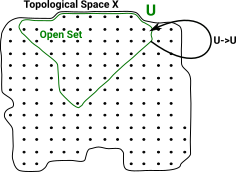and composition (U->V)*(V ->W) = U->W (composition)For a non-category theory view of this structure see page here.

## Bn - Category of Bundles

 A fibre bundle is a function f:(A->I) This is described on the following pages: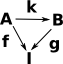We can make this a category where: objects are pairs (A,f) consisting of a stalk 'A' and a mapping of 'A' to 'I'. morphisms are functors between these where I does not change. This triangle must commute. So the elements (germs) of a stalk in 'A' must map to the same stalk in 'B'. This is a comma category as discussed on this page.The Bn Category can lead on to the concept of a Topos.

## Simplical Sets

We have looked at these, mostly from a topology point of view, on the pages here:

The subject can also be approached from a purely combinatorial point of view.

Here we investigate how these structures can be viewed in a category theory way.

 Δ is a category with: objects - are ordered sets which represent simplicies. morphisms - are order preserving maps. The morphisms are inclusions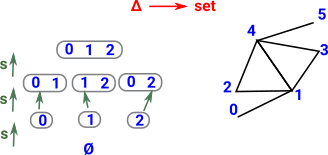Δop is a category with the same objects but morphisms are the face maps.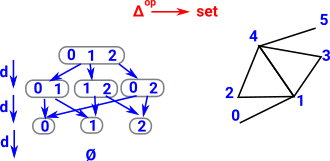## Category of Presheaves

### Objects: Cop -> set

contraveriant functors X: C -> set

(written X: Cop -> set to indicate contraveriance)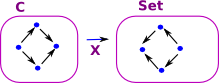### Morphisms:

are natural transformations N: X -> Y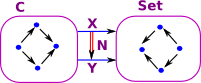For a non-category theory view of this structure see page here.